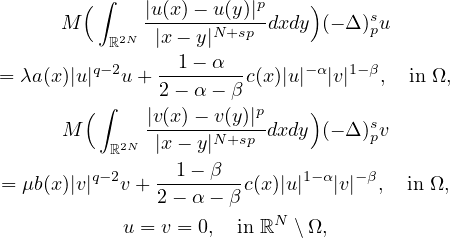Electron. J. Differential Equations, Vol. 2022 (2022), No. 77, pp. 1-15.

### Kirchhoff systems involving fractional p-Laplacian and singular nonlinearity Mouna Kratou

Abstract:
In this work we consider the fractional Kirchhoff equations with singular nonlinearity,where Ω is a bounded domain in RN with smooth boundary, N> ps, s in (0,1), 0<α<1, 0< β< 1, 2-α-β<p≤ pθ<q<p*s, p*s=2N/(N-2s) is the fractional Sobolev exponent, λ, μ are two parameters, a, b, c in C(overlineΩ) are non-negative weight functions, M(t)=k+ltθ-1 with k>0, l,θ≥1, and (-Δ)sp is the fractional p-laplacian operator. We prove the existence of multiple non-negative solutions by studying the nature of the Nehari manifold with respect to the parameters λ and μ.

Submitted September 11, 2022. Published November 21, 2022.
Math Subject Classifications: 34B15, 37C25, 35R20.
Key Words: Kirchhoff-type equations; fractional p-Laplace operator; Nehari manifold; singular elliptic system; multiple positive solutions,

Show me the PDF file (387 KB), TEX file for this article.

 Mouna Kratou College of sciences at Dammam University of Imam Abdulrahman Bin Faisal 31441 Dammam, Saudi Arabia email: mmkratou@iau.edu.sa